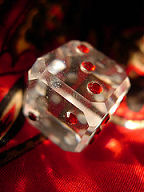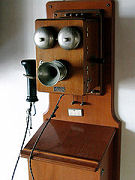Search IntMath
Close

450+ Math Lessons written by Math Professors and Teachers

5 Million+ Students Helped Each Year

1200+ Articles Written by Math Educators and Enthusiasts

Simplifying and Teaching Math for Over 23 Years

# 12. The Binomial Probability Distribution

### Notation

We use upper case variables (like X and Z) to denote random variables, and lower-case letters (like x and z) to denote specific values of those variables.

A binomial experiment is one that possesses the following properties:

1. The experiment consists of n repeated trials;

2. Each trial results in an outcome that may be classified as a success or a failure (hence the name, binomial);

3. The probability of a success, denoted by p, remains constant from trial to trial and repeated trials are independent.

The number of successes X in n trials of a binomial experiment is called a binomial random variable.

The probability distribution of the random variable X is called a binomial distribution, and is given by the formula:

P(X)=C_x^n p^x q^(n-x)

where

n = the number of trials

x = 0, 1, 2, ... n

p = the probability of success in a single trial

q = the probability of failure in a single trial

(i.e. q = 1 − p)

C_x^n is a combination

P(X) gives the probability of successes in n binomial trials.

## Mean and Variance of Binomial Distribution

If p is the probability of success and q is the probability of failure in a binomial trial, then the expected number of successes in n trials (i.e. the mean value of the binomial distribution) is

E(X) = μ = np

The variance of the binomial distribution is

V(X) = σ2 = npq

Note: In a binomial distribution, only 2 parameters, namely n and p, are needed to determine the probability.

### Example 1Image source

A die is tossed 3 times. What is the probability of

(a) No fives turning up?

(b) 1 five?

(c) 3 fives?

This is a binomial distribution because there are only 2 possible outcomes (we get a 5 or we don't).

Now, n = 3 for each part. Let X = number of fives appearing.

(a) Here, x = 0.

P(X=0) =C_x^np^xq^[n-x] =C_0^3 (1/6)^0 (5/6)^3 =125/216 =0.5787

(b) Here, x = 1.

P(X=1) =C_x^np^xq^[n-x] =C_1^3 (1/6)^1 (5/6)^2 =75/216 =0.34722

(c) Here, x = 3.

P(X=3)=C_x^np^xq^[n-x] =C_3^3 (1/6)^3 (5/6)^0 =1/216 =4.6296times10^-3

### Example 2

Hospital records show that of patients suffering from a certain disease, 75% die of it. What is the probability that of 6 randomly selected patients, 4 will recover?

This is a binomial distribution because there are only 2 outcomes (the patient dies, or does not).

Let X = number who recover.

Here, n = 6 and x = 4. Let p = 0.25 (success, that is, they live), q = 0.75 (failure, i.e. they die).

The probability that 4 will recover:

P(X) = C_x^np^xq^[n-x] =C_4^6(0.25)^4(0.75)^2 =15times 2.1973 times 10^-3 =0.0329595

#### Histogram of this distribution:

We could calculate all the probabilities involved and we would get:

 X text[Probability] 0 0.17798 1 0.35596 2 0.29663 3 0.13184 4 3.2959 times 10^-2 5 4.3945times10^-3 6 2.4414times10^-4

The histogram is as follows:

It means that out of the 6 patients chosen, the probability that:

• None of them will recover is 0.17798,
• One will recover is 0.35596, and
• All 6 will recover is extremely small.

### Example 3Image source

In the old days, there was a probability of 0.8 of success in any attempt to make a telephone call. (This often depended on the importance of the person making the call, or the operator's curiosity!)

Calculate the probability of having 7 successes in 10 attempts.

Probability of success p = 0.8, so q = 0.2.

X = success in getting through.

Probability of 7 successes in 10 attempts:

text[Probability]=P(X=7)

=C_7^10(0.8)^7(0.2)^[10-7]

=0.20133

#### Histogram

We use the following function

C(10,x)(0.8)^x(0.2)^[10-x]

to obtain the probability histogram:

### Example 4

A (blindfolded) marksman finds that on the average he hits the target 4 times out of 5. If he fires 4 shots, what is the probability of

(a) more than 2 hits?

(b) at least 3 misses?

Here, n = 4, p = 0.8, q = 0.2.

Let X = number of hits.

Let x0 = no hits, x1 = 1 hit, x2 = 2 hits, etc.

(a) P(X)=P(x_3)+P(x_4)

=C_3^4(0.8)^3(0.2)^1+ C_4^4(0.8)^4(0.2)^0

=4(0.8)^3(0.2)+(0.8)^4

=0.8192

(b) 3 misses means 1 hit, and 4 misses means 0 hits.

P(X)=P(x_1)+P(x_0)

=C_1^4(0.8)^1(0.2)^3+ C_0^4(0.8)^0(0.2)^4

=4(0.8)^1(0.2)^3+(0.2)^4

=0.0272

### Example 5Image source

The ratio of boys to girls at birth in Singapore is quite high at 1.09:1.

What proportion of Singapore families with exactly 6 children will have at least 3 boys? (Ignore the probability of multiple births.)

[Interesting and disturbing trivia: In most countries the ratio of boys to girls is about 1.04:1, but in China it is 1.15:1.]

The probability of getting a boy is 1.09/(1.09+1.00)=0.5215

Let X = number of boys in the family.

Here,

n = 6,
p = 0.5215,
q = 1 − 0.52153 = 0.4785

When x=3:

 P(X) =C_x^np^xq^(n-x) =C_3^6(0.5215)^3(0.4785)^3 =0.31077

When x=4:

 P(X) =C_4^6(0.5215)^4(0.4785)^2 =0.25402

When x=5:

P(X) =C_5^6(0.5215)^5(0.4785)^1 =0.11074

When x=6:

P(X) =C_6^6(0.5215)^6(0.4785)^0 =2.0115xx10^-2

So the probability of getting at least 3 boys is:

"Probability"=P(X>=3)

=0.31077+0.25402+ 0.11074+ 2.0115xx10^-2

=0.69565

NOTE: We could have calculated it like this:

P(X>=3) =1-(P(x_0)+P(x_1)+P(x_2))

### Example 6

A manufacturer of metal pistons finds that on the average, 12% of his pistons are rejected because they are either oversize or undersize. What is the probability that a batch of 10 pistons will contain

(a) no more than 2 rejects? (b) at least 2 rejects?

Let X = number of rejected pistons

(In this case, "success" means rejection!)

Here, n = 10, p = 0.12, q = 0.88.

(a)

No rejects. That is, when x=0:

P(X) =C_x^np^xq^(n-x) =C_0^10(0.12)^0(0.88)^10 =0.2785

One reject. That is, when x=1

P(X) =C_1^10(0.12)^1(0.88)^9 =0.37977

Two rejects. That is, when x=2:

P(X) =C_2^10(0.12)^2(0.88)^8 =0.23304

So the probability of getting no more than 2 rejects is:

"Probability"=P(X<=2)

=0.2785+ 0.37977+ 0.23304

=0.89131

(b) We could work out all the cases for X = 2, 3, 4, ..., 10, but it is much easier to proceed as follows:

"Probablity of at least 2 rejects"

=1-P(X<=1)

 =1-(P(x_0)+P(x_1))

 =1-(0.2785+0.37977)

=0.34173

#### Histogram

Using the function g(x)=C(10,x)(0.12)^x(0.88)^(10-x) and finding the values at 0, 1, 2, ..., gives us the histogram:

## Problem SolverThis tool combines the power of mathematical computation engine that excels at solving mathematical formulas with the power of GPT large language models to parse and generate natural language. This creates math problem solver thats more accurate than ChatGPT, more flexible than a calculator, and faster answers than a human tutor. Learn More.